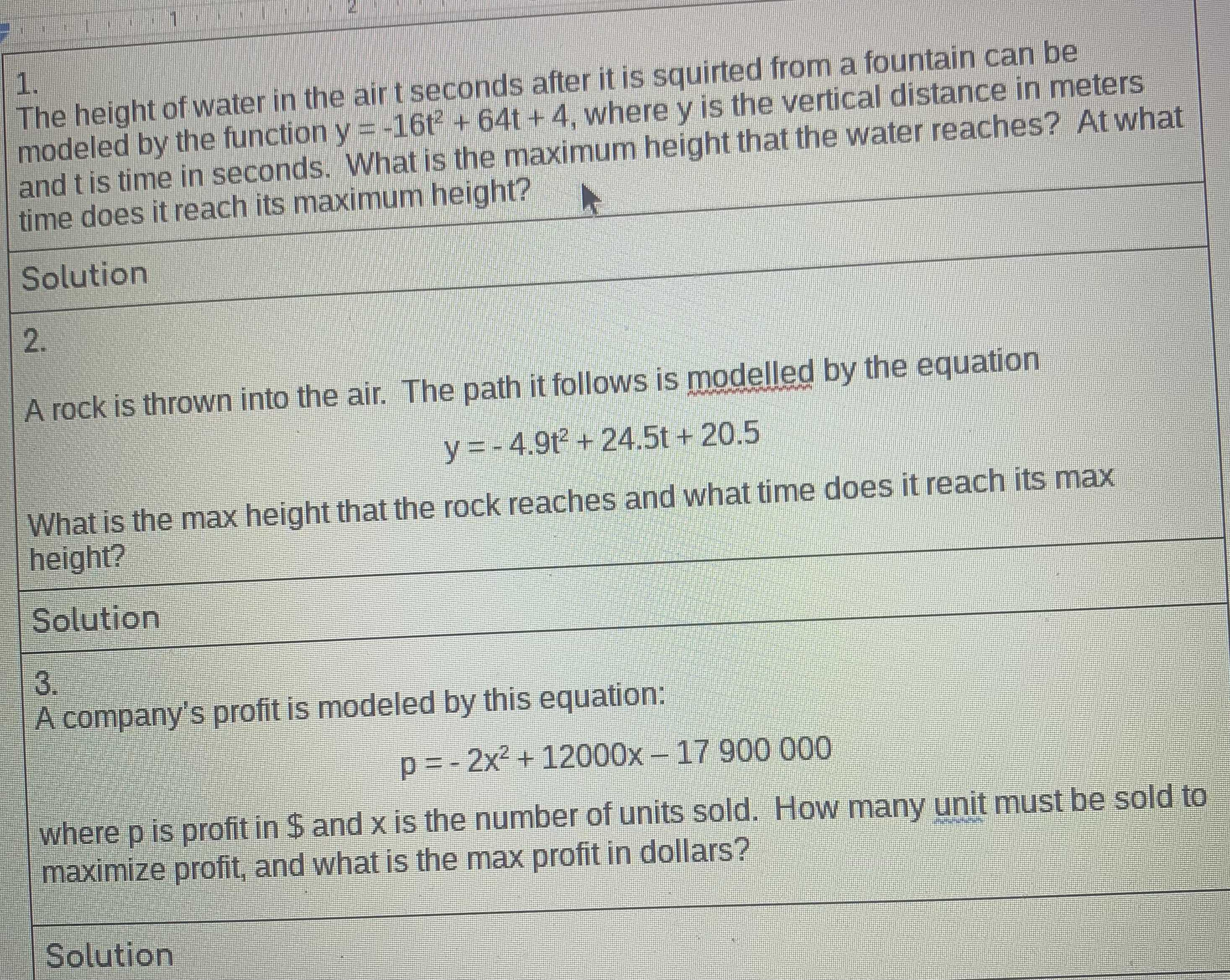### ¿Todavía tienes preguntas de matemáticas?

Pregunte a nuestros tutores expertos
Algebra
Pregunta1. The height of water in the air $$t$$ seconds after it is squirted from a fountain can be modeled by the function $$y = - 16 t ^ { 2 } + 64 t + 4$$ , where $$y$$ is the vertical distance in meters and $$t$$ is time in seconds. What is the maximum height that the water reaches? At what time does it reach its maximum height? A rock is thrown into the air. The path it follows is modelled by the equation

$$y = - 4.9 t ^ { 2 } + 24.5 t + 20.5$$ What is the max height that the rock reaches and what time does it reach its max height?

$$Solution$$ A. company's profit is modeled by this equation:

$$p = - 2 x ^ { 2 } + 12000 x - 17900000$$ where $$p$$ is profit in $$\$$ and $$x$$ is the number of units sold. How many unit must be sold to maximize profit, and what is the max profit in dollars?

1. $$y_{\max}= 4- \frac{64^{2}}{4(- 16)} = 68$$

$$t= - \frac{64}{2(- 16)} = 2$$

2. $$y_{\max}= 20.5- \frac{24.5^{2}}{4(- 4.9)} = 21.75$$

$$t= - \frac{24.5}{2(- 4.9)} = 2.5$$

3. $$p_{\max}= - 17900000- \frac{12000^{2}}{4(- 2)} = 100000$$

$$x= - \frac{12000}{2(- 2)} = 3000$$

Solución
View full explanation on CameraMath App.